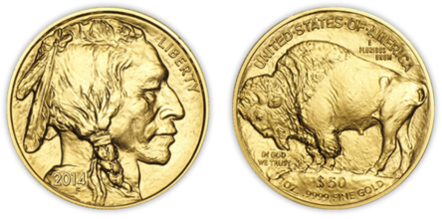# Fair Result With Biased Coin

|Here’s a nice short question: How can you get fair results from tossing a biased coin? (but not completely biased though, with a side having 0 probability!). The solution given by von Neumann is as follows:

• Toss the coin twice.
• If the results match, start over, forgetting both results.
• If the results differ, use the first result, forgetting the second.

This produces the correct result because even with this biased coin, the probability of heads then tails is equal to the probability of getting tails then heads. So out of the 4 possible events (H-T, H-H, T-H, T-T) we are left with 2 events with the same probability.

I made a small python script to see how many tries are needed as the probability of the biased faces varies away from 0.5:

``````# Biased coin probability to get heads
p = 0.7

# Simulate the bias
N = 1000000
random.seed()
v =  * int(N * p) +  * int(N * (1-p))
random.shuffle(v)

# Fair events
cnt = 0
# Total events
ev = 0

while cnt<200:
idx1 = random.randint(0, N-1)
idx2 = random.randint(0, N-1)

if(v[idx1] != v[idx2]):
cnt +=1
ev += 1

# For p = 0.5 ev ~= cnt*2
print "Events needed to get X fair tosses: %d" % (ev)
``````